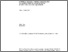# Optimal Trajectories Associated to a Solution of Contingent Hamilton-Jacobi Equation

Frankowska, H. (1987). Optimal Trajectories Associated to a Solution of Contingent Hamilton-Jacobi Equation. IIASA Working Paper. IIASA, Laxenburg, Austria: WP-87-069Preview
Text
WP-87-069.pdf

## Abstract

In this paper we study the existence of optimal trajectories associated with a generalized solution to Hamilton-Jacobi-Bellman equation arising in optimal control. In general, we cannot expect such solutions to be differentiable. But, in a way analogous to the use of distributions in PDE, we replace the usual derivatives with "contingent epiderivatives" and the Hamilton-Jacobi equation by two "contingent Hamilton-Jacobi inequalities". We show that the value function of an optimal control problem verifies these "contingent inequalities".

Our approach allows the following three results: (1) The upper semicontinuous solutions to contingent inequalities are monotone along the trajectories of the dynamical system. (2) With every continuous solution V of the contingent inequalities, we can associate an optimal trajectory along which V is constant. (3) For such solutions, we can construct optimal trajectories through the corresponding optimal feedback.

They are also "viscosity solutions" of a Hamilton-Jacobi equation. Finally we prove a relationship between super-differentials of solutions introduced in Crandall-Evans-Lions and the Pontryagin principle and discuss the link of viscosity solutions with Clarke's approach to the Hamilton-Jacobi equation.

Item Type: Monograph (IIASA Working Paper) System and Decision Sciences - Core (SDS) IIASA Import 15 Jan 2016 01:57 27 Aug 2021 17:13 http://pure.iiasa.ac.at/2983View Item